# gateR: Flow/Mass Cytometry Gating via Spatial Kernel Density Estimation

#### 2023-02-01

The gateR package is a suite of R functions to identify significant spatial clustering of mass and flow cytometry data used in immunological investigations. The gateR package can be used for a panel of all surface markers or a mixture of surface markers and functional readouts. The gateR package performs a gating technique that estimates statistically significant marker combination values within which one immunologically distinctive group (i.e., disease case) is more associated than another group (i.e., healthy control), successively, using various combinations (i.e., “gates”) of markers to examine features of cells that may be different between groups. For a two-group comparison, the gateR package uses the spatial relative risk function estimated using the sparr package. The gates are conducted in two-dimensional space comprised of two markers.

Examples of a single condition with two groups:

1. Disease case vs. Healthy control
2. Time 2 vs. Time 1 (baseline)

For a two-group comparison of two conditions, we estimate two relative risk surfaces for one condition and then a ratio of the relative risks. For example:

1. Estimate a relative risk surface for:
1. Condition 2B vs. Condition 2A
2. Condition 1B vs. Condition 1A
2. Estimate the relative risk surface for the ratio:

$\frac{(\frac{Condition2B}{Condition2A})}{(\frac{Condition1B}{Condition1A})}$

Within areas where the relative risk exceeds an asymptotic normal assumption, the gateR package has the functionality to examine the features of these cells.

This vignette implements the gateR package using a randomly generated data set. Please see the README.md file within the gateR GitHub repository for an example using publicly available flow cytometry data from the flowWorkspaceData package available via Bioconductor. Here, we generate data with two conditions, four markers, and two additional features.

  loadedPackages <- c("gateR", "graphics", "stats", "tibble", "utils")
set.seed(1234) # for reproducibility

### Generate random toy data

A unique function randomly generates multivariate normal (MVN) data around a central point. Parameters include the centroid coordinates (centre), the number of observations to generate (ncell), and the standard deviation of the normal distribution (scalar).

  rand_mvn <- function(centre, ncell, scalar) {
x0 <- centre
y0 <- centre
x1 <- rep(x0, ncell)
y1 <- rep(y0, ncell)
x2 <- x1 + stats::rnorm(ncell, 0, scalar)
y2 <- y1 + stats::rnorm(ncell, 0, scalar)
x <- cbind(x2, y2)
}

#### Gate 1: Marker 1 and Marker 2

At Condition 1, we generate 100,000 cases and 100,000 controls (ncell = 100000) randomly MVN with a case centroid at (0.55, 0.55) and a control centroid at (0.40, 0.40) within a unit square window (0, 1), and cases have a more focal cluster (scalar = 0.05) than controls (scalar = 0.15).

# Initial parameters
ncell <- 100000 # number of observations per group per condition
c1_cas_center <- c(0.55, 0.55)
c1_con_center <- c(0.40, 0.40)
# V1 and V2 at Condition 1
c1_cas <- rand_mvn(centre = c1_cas_center, ncell = ncell, scalar = 0.05)
c1_con <- rand_mvn(centre = c1_con_center, ncell = ncell, scalar = 0.15)
graphics::par(pty = "s")
graphics::plot(c1_con,
col = "blue",
xlim = c(0, 1),
ylim = c(0, 1),
main = "Gate 1, Condition 1",
xlab = "V1",
ylab = "V2")
graphics::points(c1_cas, col = "orangered4")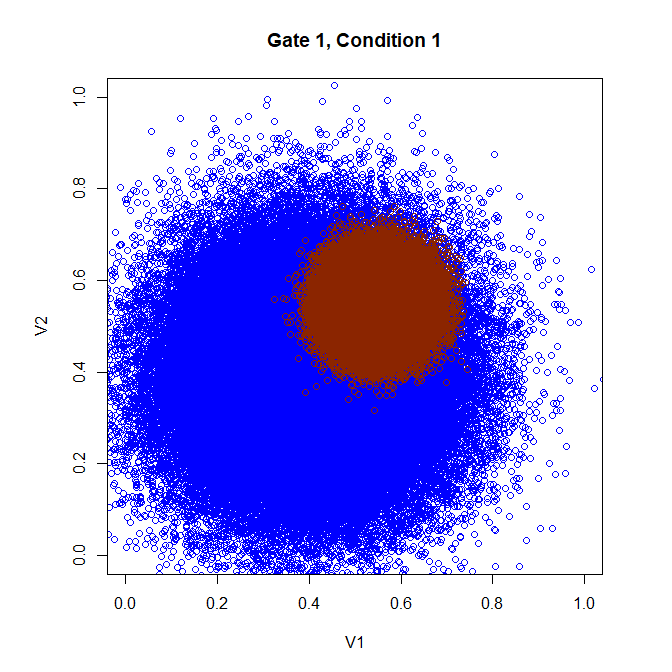At Condition 2, we generate 100,000 cases and 100,000 controls (ncell = 100000) randomly MVN with a case centroid at (0.45, 0.45) and a control centroid at (0.40, 0.40) within a unit square window (0, 1), and cases have a more focal cluster (scalar = 0.05) than controls (scalar = 0.10).

# Initial parameters
c2_cas_center <- c(0.45, 0.45)
c2_con_center <- c(0.40, 0.40)
# V1 and V2 at Condition 2
c2_cas <- rand_mvn(centre = c2_cas_center, ncell = ncell, scalar = 0.05)
c2_con <- rand_mvn(centre = c2_con_center, ncell = ncell, scalar = 0.10)
graphics::par(pty = "s")
graphics::plot(c2_con,
col = "cornflowerblue",
xlim = c(0, 1),
ylim = c(0, 1),
main = "Gate 1, Condition 2",
xlab = "V1",
ylab = "V2")
graphics::points(c2_cas, col = "orangered1")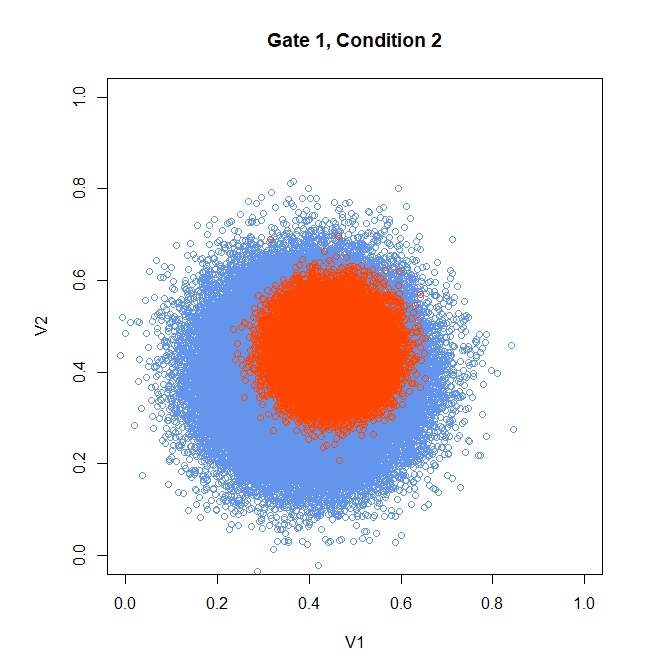# compile data
df_full <- tibble::tibble("id" = seq(1, ncell * 2 * 2, 1),
"group" = factor(c(rep("case", ncell * 2),
rep("control", ncell * 2))),
"condition" = factor(c(rep("2", ncell), rep("1", ncell),
rep("2", ncell), rep("1", ncell))),
"V1" = c(c2_cas[ , 1], c1_cas[ , 1], c2_con[ , 1], c1_con[ , 1]),
"V2" = c(c2_cas[ , 2], c1_cas[ , 2], c2_con[ , 2], c1_con[ , 2]))

rm(c2_cas, c1_cas, c2_con, c1_con) # conserve memory

#### Gate 2: Marker 3 and Marker 4

At Condition 1, we generate 100,000 cases and 100,000 controls (ncell = 100000) randomly MVN with a case centroid at (0.55, 0.55) and a control centroid at (0.50, 0.50) within a unit square window (0, 05), but both have the same amount of spread (scalar = 0.10).

# Initial parameters
c1_cas_center <- c(0.55, 0.55)
c1_con_center <- c(0.50, 0.50)
# V3 and V4 at Condition 1
c1_cas <- rand_mvn(centre = c1_cas_center, ncell = ncell, scalar = 0.05)
c1_con <- rand_mvn(centre = c1_con_center, ncell = ncell, scalar = 0.10)
graphics::par(pty = "s")
graphics::plot(c1_con,
col = "blue",
xlim = c(0, 1),
ylim = c(0, 1),
main = "Gate 2, Condition 1",
xlab = "V3",
ylab = "V4")
graphics::points(c1_cas, col = "orangered4")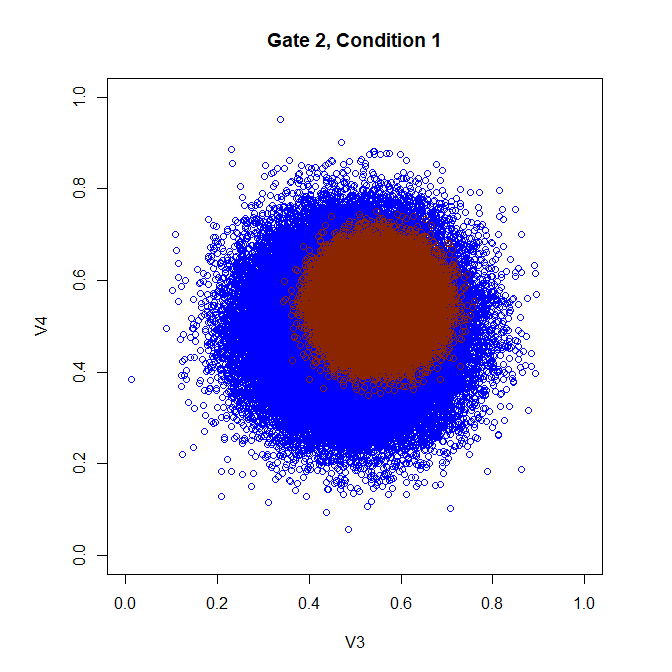At Condition 2, we generate 100,000 cases and 100,000 controls (ncell = 100000) randomly with a case centroid at (0.65, 0.65) and control a centroid at (0.50, 0.50) within a unit square window (0, 1), and cases have a more focal cluster (scalar = 0.05) than controls (scalar = 0.10).

# Initial parameters
c2_cas_center <- c(0.65, 0.65)
c2_con_center <- c(0.50, 0.50)
# V3 and V4 at Condition 2
c2_cas <- rand_mvn(centre = c2_cas_center, ncell = ncell, scalar = 0.05)
c2_con <- rand_mvn(centre = c2_con_center, ncell = ncell, scalar = 0.10)
graphics::par(pty = "s")
graphics::plot(c2_con,
col = "cornflowerblue",
xlim = c(0, 1),
ylim = c(0, 1),
main = "Gate 2, Condition 2",
xlab = "V3",
ylab = "V4")
graphics::points(c2_cas, col = "orangered1")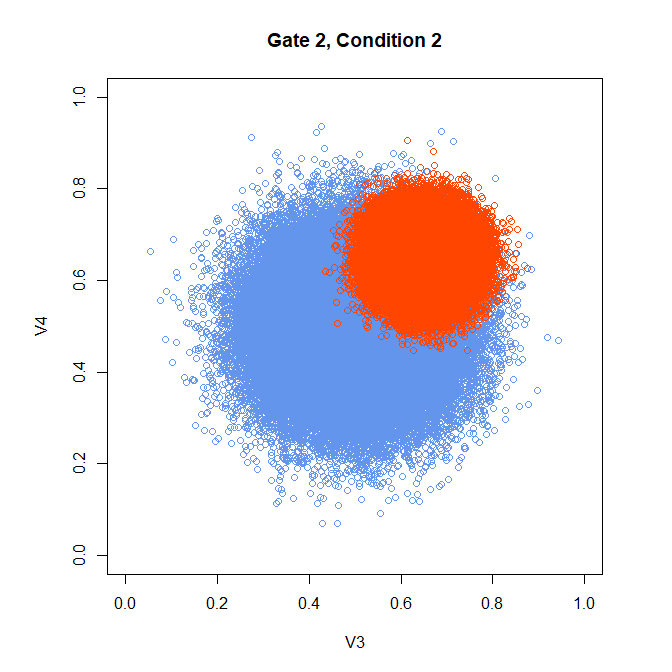Compile the toy data into a data frame

  df_full$V3 <- c(c2_cas[ , 1], c1_cas[ , 1], c2_con[ , 1], c1_con[ , 1]) df_full$V4 <-  c(c2_cas[ , 2], c1_cas[ , 2], c2_con[ , 2], c1_con[ , 2])

rm(c2_cas, c1_cas, c2_con, c1_con) # conserve memory

Generate random values for two example cytokines and append to the data frame.

# Two Cytokines
Z1 <- stats::rchisq(ncell * 4, df = 5) # Random Chi-square distribution
Z2 <- stats::rnorm(ncell * 4, 0, 1) # Random Gaussian distribution
# Append to data.frame
df_full$Z1 <- Z1 df_full$Z2 <- Z2
rm(Z1, Z2) # conserve memory
# Visualize histograms by the two group conditions
graphics::par(mfrow = c(2, 2), pty = "s")
graphics::plot(stats::density(df_full$Z1[df_full$group == "case"
& df_full$condition == "1"]), main = "Cytokine 1 of Cases at Condition 1") graphics::plot(stats::density(df_full$Z1[df_full$group == "case" & df_full$condition == "2"]),
main = "Cytokine 1 of Cases at Condition 2")
graphics::plot(stats::density(df_full$Z1[df_full$group == "control"
& df_full$condition == "1"]), main = "Cytokine 1 of Controls at Condition 1") graphics::plot(stats::density(df_full$Z1[df_full$group == "control" & df_full$condition == "2"]),
main = "Cytokine 1 of Controls at Condition 2")
graphics::plot(stats::density(df_full$Z2[df_full$group == "case"
& df_full$condition == "1"]), main = "Cytokine 2 of Cases at Condition 1") graphics::plot(stats::density(df_full$Z2[df_full$group == "case" & df_full$condition == "2"]),
main = "Cytokine 2 of Cases at Condition 2")
graphics::plot(stats::density(df_full$Z2[df_full$group == "control"
& df_full$condition == "1"]), main = "Cytokine 2 of Controls at Condition 1") graphics::plot(stats::density(df_full$Z2[df_full$group == "control" & df_full$condition == "2"]),
main = "Cytokine 2 of Controls at Condition 2")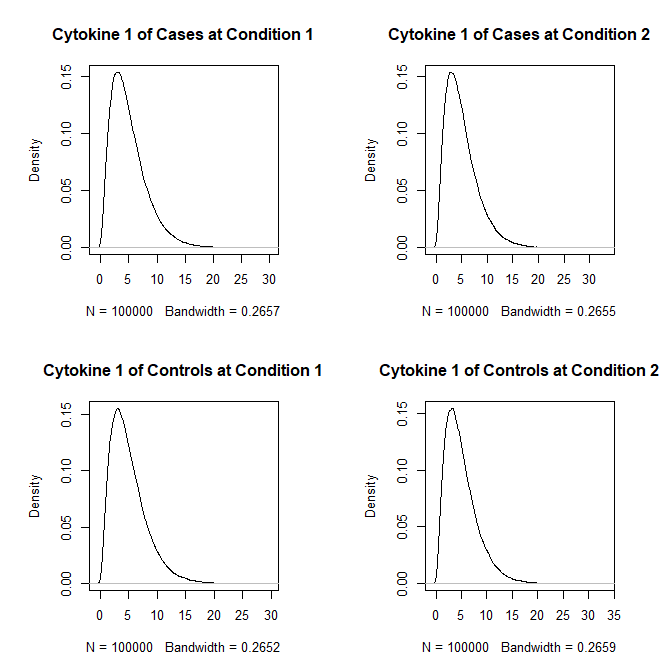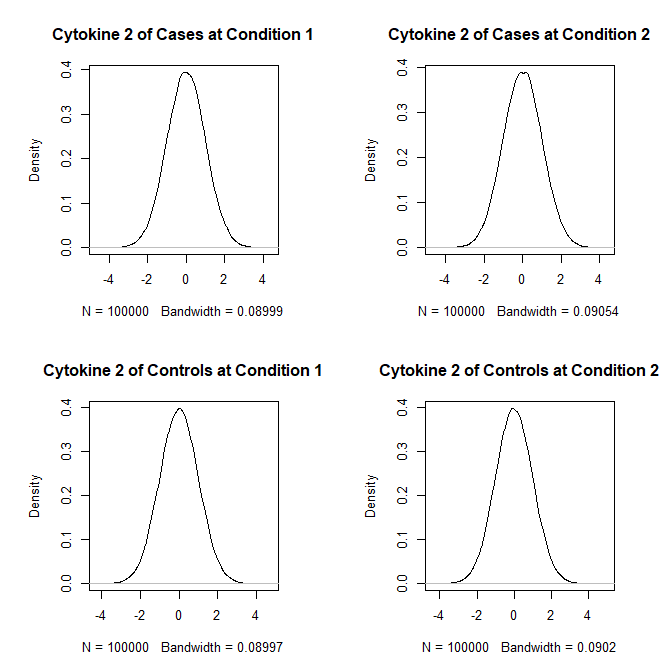The toy data frame has nine columns (id, groups, markers, and cytokines).

  utils::head(df_full)
## # A tibble: 6 × 9
##      id group condition    V1    V2    V3    V4    Z1      Z2
##   <dbl> <fct> <fct>     <dbl> <dbl> <dbl> <dbl> <dbl>   <dbl>
## 1     1 case  2         0.491 0.402 0.677 0.586  4.35 -0.488
## 2     2 case  2         0.407 0.493 0.714 0.698  8.61  0.279
## 3     3 case  2         0.508 0.409 0.547 0.644  6.79 -0.786
## 4     4 case  2         0.423 0.480 0.657 0.656  1.04 -0.552
## 5     5 case  2         0.367 0.420 0.635 0.637  4.10  0.239
## 6     6 case  2         0.499 0.405 0.547 0.656  6.99  0.0472

### For two conditions

# Initial parameters
alpha <- 0.05
vars <- c("V1", "V2", "V3", "V4")
p_correct <- "correlated Bonferroni"
set.seed(1234) # for reproducibility
df_full <- as.data.frame(df_full)

# Gates 1 and 2
start_time <- Sys.time() # record start time
out_gate <- gateR::gating(dat = df_full,
vars = vars,
n_condition = 2,
plot_gate = TRUE,
alpha = alpha,
p_correct = p_correct,
c1n = "case", # level "case" as the numerator of first condition
c2n = "2") # level "2" as the numerator of second condition
end_time <- Sys.time() # record end time
total_time <- end_time - start_time # calculate duration of gating() example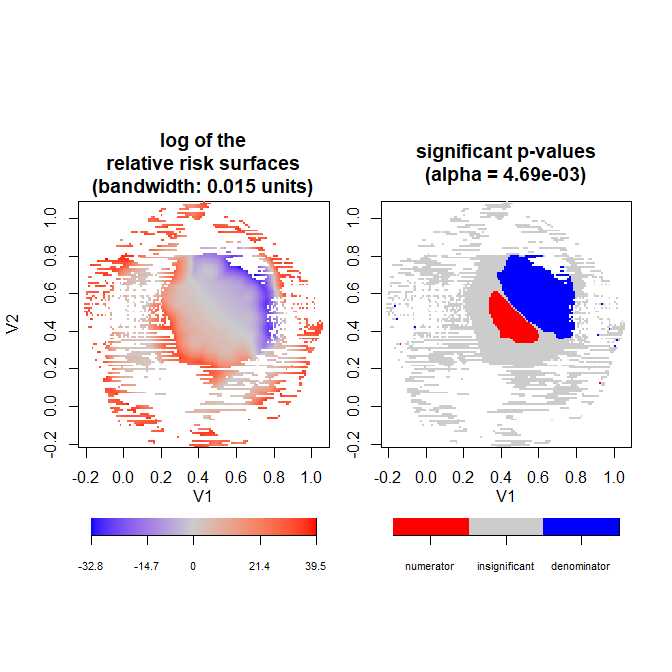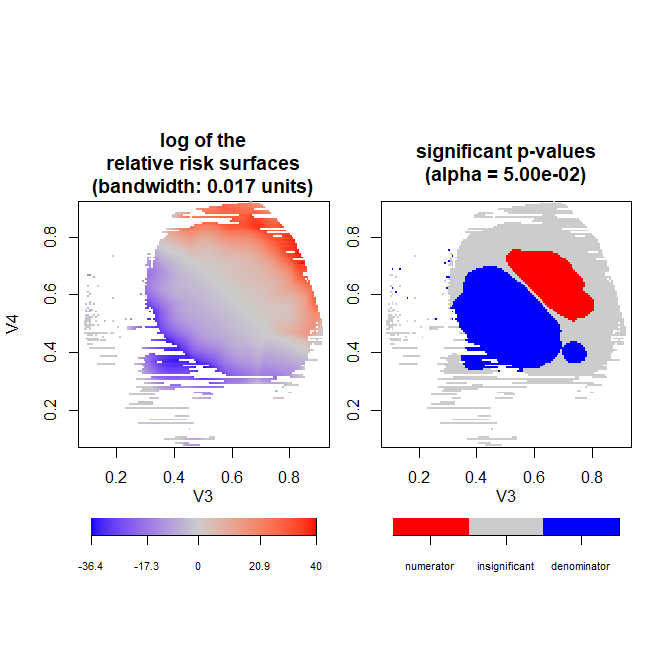The gating process took about 19.1 seconds on a machine with the features listed at the end of the vignette (4 variables, 2 gates, 2 cytokines, 400,000 observations). The corrected significance level in the first gate was . The histograms for the two cytokines are the same as above.

# Plot of Cytokine 1
graphics::par(mfrow = c(1, 2), pty = "s")
graphics::plot(stats::density(out_gate$obs$Z1[out_gate$obs$group == "case"
& out_gate$obs$condition == "2"]),
col = "red", main = "Cytokine 1 of cases\npost-gating",
xlim = c(-5, 30),
ylim = c(0, 0.2))
graphics::plot(stats::density(out_gate$obs$Z1[out_gate$obs$group == "control"
& out_gate$obs$condition == "2"]),
col = "blue",
main = "Cytokine 1 of controls\npost-gating",
xlim = c(-5, 30),
ylim = c(0, 0.2))
# Plot of Cytokine 2
graphics::par(mfrow = c(1, 2), pty = "s")
graphics::plot(stats::density(out_gate$obs$Z2[out_gate$obs$group == "case"
& out_gate$obs$condition == "2"]),
col = "red",
main = "Cytokine 2 of cases\npost-gating",
xlim = c(-5, 5),
ylim = c(0, 0.5))
graphics::plot(stats::density(out_gate$obs$Z2[out_gate$obs$group == "control"
& out_gate$obs$condition == "2"]),
col = "blue",
main = "Cytokine 2 of controls\npost-gating",
xlim = c(-5, 5),
ylim = c(0, 0.5))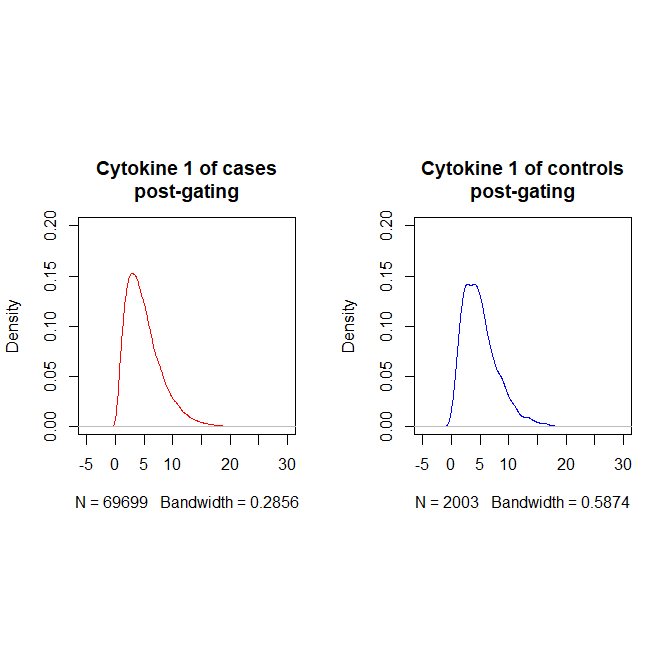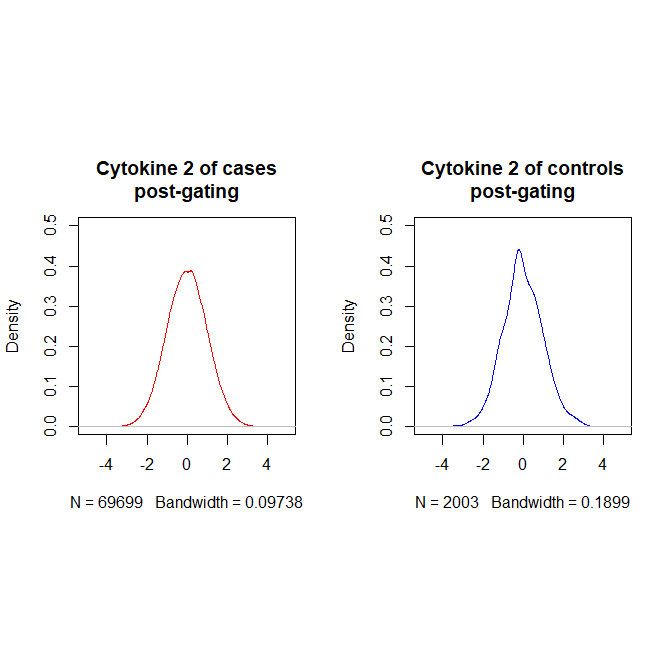Compare histograms before and after gating. Gating reduced the overall sample size of observations from 400,000 (cases & controls and Condition 1 & Condition 2) to 73,316 observations (cases & controls and Condition 1 & Condition 2).

# Plot of Cytokine 1
graphics::par(mfrow = c(1, 2), pty = "s")
graphics::plot(stats::density(df_full$Z1[df_full$group == "case"
& df_full$condition == "2"]), col = "black", lty = 1, main = "Cytokine 1 of cases\npre-gating", xlim = c(-5, 30), ylim = c(0, 0.2)) graphics::plot(stats::density(out_gate$obs$Z1[out_gate$obs$group == "case" & out_gate$obs$condition == "2"]), col = "black", lty = 1, main = "Cytokine 1 of cases\npost-gating", xlim = c(-5, 30), ylim = c(0, 0.2)) # Plot of Cytokine 2 graphics::par(mfrow = c(1, 2), pty = "s") graphics::plot(stats::density(df_full$Z2[df_full$group == "case" & df_full$condition == "2"]),
col = "black",
lty = 1,
main = "Cytokine 2 of cases\npre-gating",
xlim = c(-5, 5),
ylim = c(0, 0.5))
graphics::plot(stats::density(out_gate$obs$Z2[out_gate$obs$group == "case"
& out_gate$obs$condition == "2"]),
col = "black",
lty = 1,
main = "Cytokine 2 of cases\npost-gating",
xlim = c(-5, 5),
ylim = c(0, 0.5))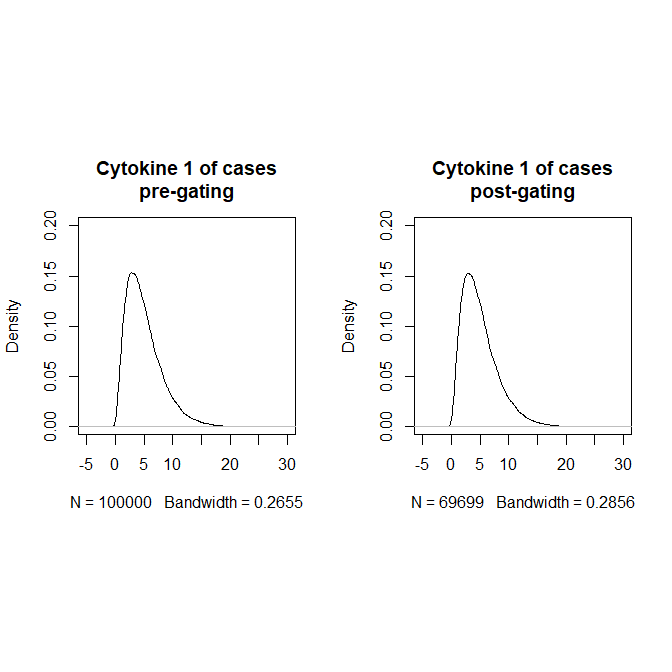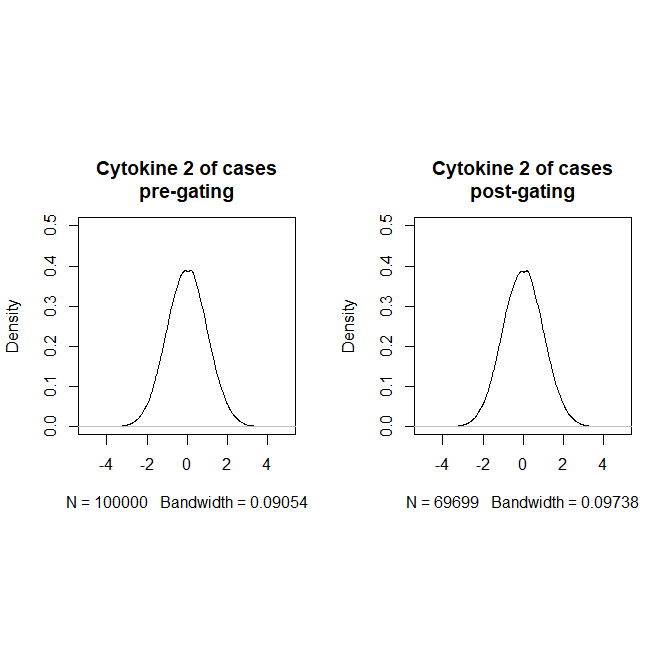### For a one condition (using only Condition 1)

# Data subset, only c1
df_sub <- df_full[df_full$condition == 1, ] # For only condition condition = 1 # Initial parameters alpha <- 0.05 vars <- c("V1", "V2", "V3", "V4") p_correct <- "correlated Bonferroni" set.seed(1234) # for reproducibility # Gates 1 and 2 start_time <- Sys.time() # record start time out_gate <- gateR::gating(dat = df_sub, vars = vars, plot_gate = TRUE, n_condition = 1, alpha = alpha, p_correct = p_correct, c1n = "case") # level "case" as the numerator of first condition end_time <- Sys.time() # record end time total_time <- end_time - start_time # calculate duration of gating() example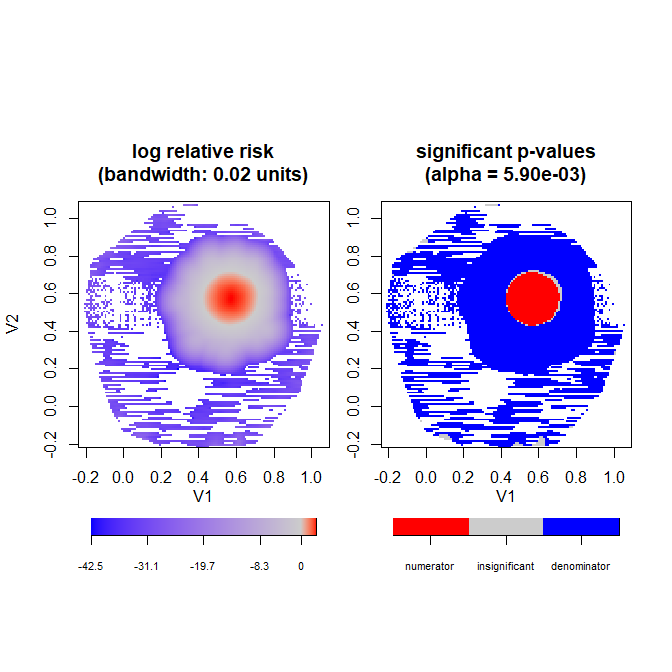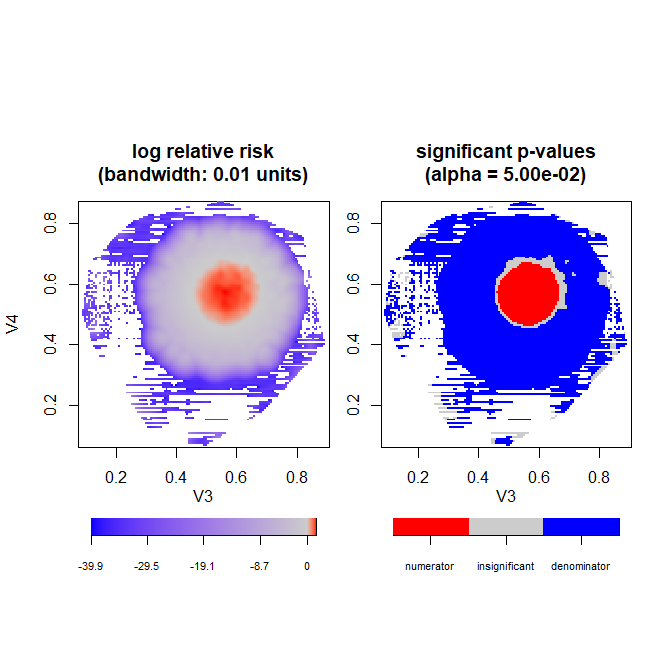The gating process took about 22.8 seconds on a machine with the features listed at the end of the vignette (4 variables, 2 gates, 2 cytokines, 200,000 observations). The corrected significance level in the first gate was . The histograms for the two cytokines are the same as above. # Plot of Cytokine 1 graphics::par(mfrow = c(1, 2), pty = "s") graphics::plot(stats::density(out_gate$obs$Z1[out_gate$obs$group == "case"]), col = "red", main = "Cytokine 1 of cases\npost-gating", xlim = c(-5, 30), ylim = c(0, 0.2)) graphics::plot(stats::density(out_gate$obs$Z1[out_gate$obs$group == "control"]), col = "blue", main = "Cytokine 1 of controls\npost-gating", xlim = c(-5, 30), ylim = c(0, 0.2)) # Plot of Cytokine 2 graphics::par(mfrow = c(1, 2), pty = "s") graphics::plot(stats::density(out_gate$obs$Z2[out_gate$obs$group == "case"]), col = "red", main = "Cytokine 2 of cases\npost-gating", xlim = c(-5, 5), ylim = c(0, 0.5)) graphics::plot(stats::density(out_gate$obs$Z2[out_gate$obs$group == "control"]), col = "blue", main = "Cytokine 2 of controls\npost-gating", xlim = c(-5, 5), ylim = c(0, 0.5))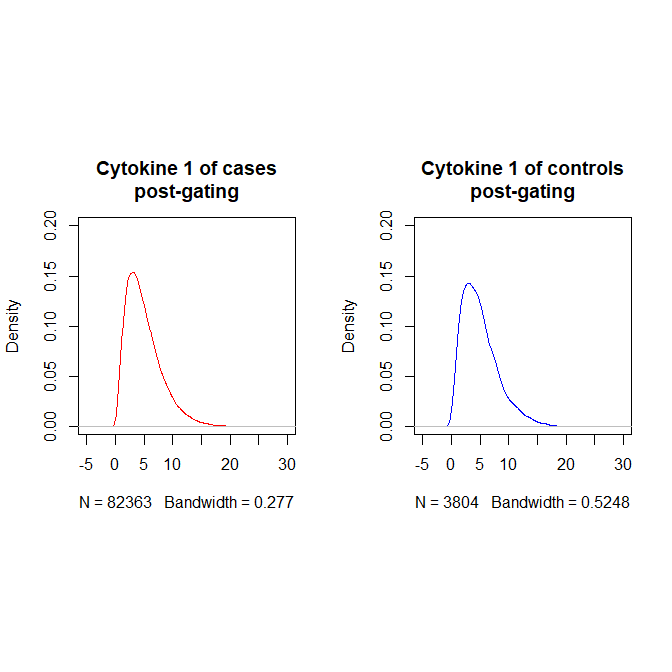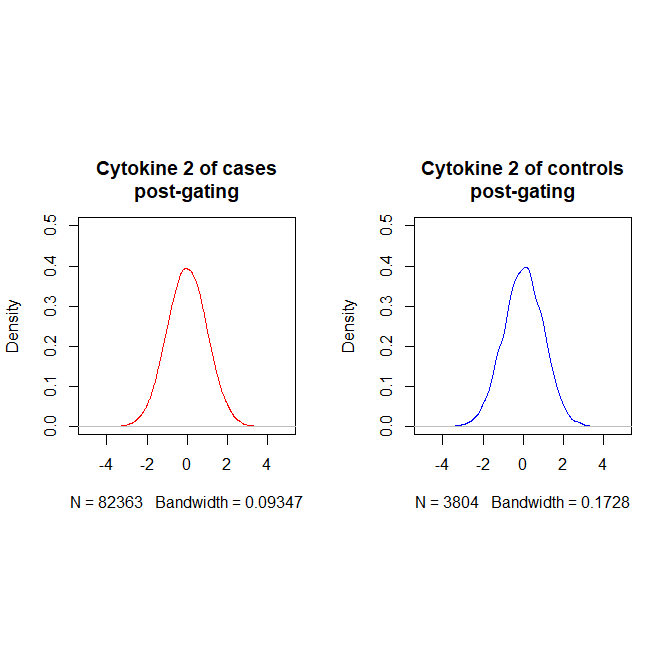Compare histograms before and after gating. Gating reduced the overall sample size of observations from 200,000 (cases & controls) to 86,167 observations (cases & controls). # Plot of Cytokine 1 graphics::par(mfrow = c(1, 2), pty = "s") graphics::plot(stats::density(df_full$Z1[df_full$group == "case"]), col = "black", lty = 1, main = "Cytokine 1 of cases\npre-gating", xlim = c(-5, 30), ylim = c(0, 0.2)) graphics::plot(stats::density(out_gate$obs$Z1[out_gate$obs$group == "case"]), col = "black", lty = 1, main = "Cytokine 1 of cases\npost-gating", xlim = c(-5, 30), ylim = c(0, 0.2)) # Plot of Cytokine 2 graphics::par(mfrow = c(1, 2), pty = "s") graphics::plot(stats::density(df_full$Z2[df_full$group == "case"]), col = "black", lty = 1, main = "Cytokine 2 of cases\npre-gating", xlim = c(-5, 5), ylim = c(0, 0.5)) graphics::plot(stats::density(out_gate$obs$Z2[out_gate$obs\$group == "case"]),
col = "black",
lty = 1,
main = "Cytokine 2 of cases\npost-gating",
xlim = c(-5, 5),
ylim = c(0, 0.5))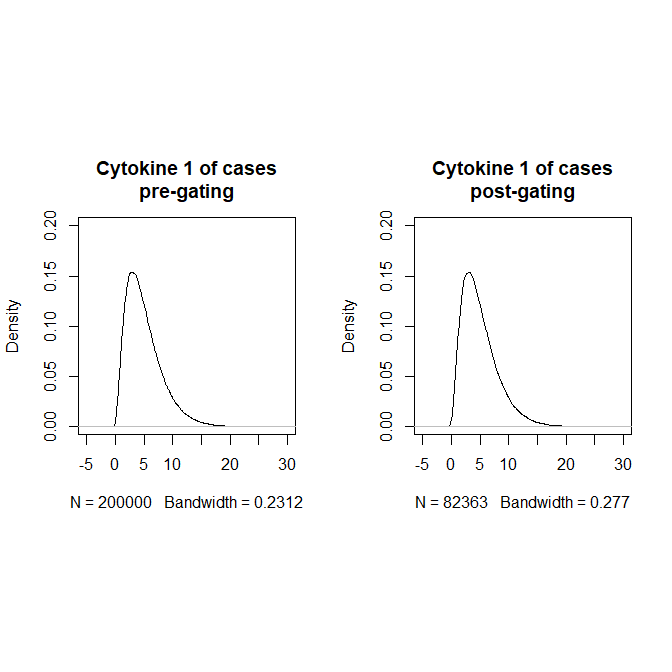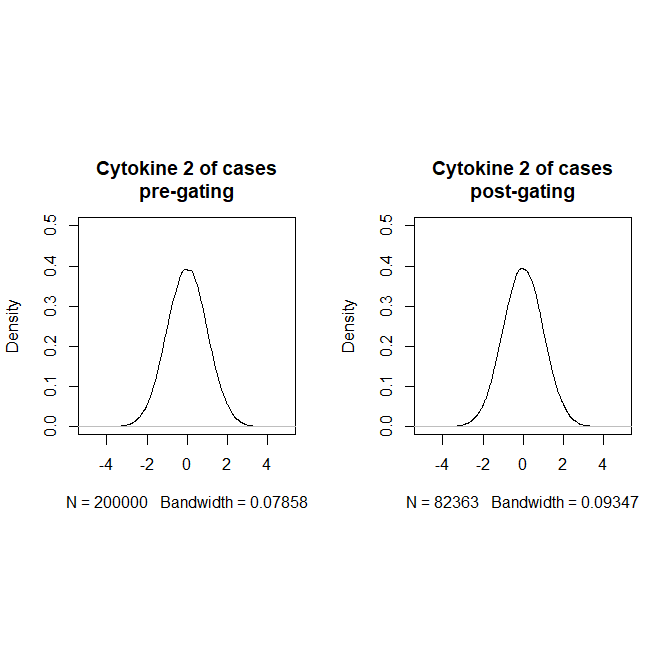### Current limitations

1. Extracts observations at all significant clusters (either case or controls), and there is currently no functionality to select cells within a specific (set of) cluster(s) for the next gate.
2. Only two dimensions (i.e., markers) per gate because the spatial relative risk function is a two-dimensional spatial statistic.
3. Only two-group comparisons (e.g., case vs. control) per gate because the spatial relative risk function is a ratio by nature.
4. Only comparisons of one condition or two conditions are possible.
5. Large computational expense (i.e., run-time) to calculate the correlated Bonferroni correction.
6. A large sample size of observations (i.e., cells) may overload the gateR process. We are evaluating this potential limitation and developing a possible solution (e.g., randomly subsetting the data to estimate the clusters at each gate).
sessionInfo()
## R version 4.2.1 (2022-06-23 ucrt)
## Platform: x86_64-w64-mingw32/x64 (64-bit)
## Running under: Windows 10 x64 (build 19045)
##
## Matrix products: default
##
## locale:
##  LC_COLLATE=English_United States.utf8
##  LC_CTYPE=English_United States.utf8
##  LC_MONETARY=English_United States.utf8
##  LC_NUMERIC=C
##  LC_TIME=English_United States.utf8
##
## attached base packages:
##  stats     graphics  grDevices utils     datasets  methods   base
##
## other attached packages:
##  tibble_3.1.8 gateR_0.1.13
##
## loaded via a namespace (and not attached):
##   Rcpp_1.0.10            lattice_0.20-45        deldir_1.0-6
##   foreach_1.5.2          digest_0.6.31          utf8_1.2.2
##   R6_2.5.1               evaluate_0.20          spam_2.9-1
##  highr_0.10             ggplot2_3.4.0          tensor_1.5
##  pillar_1.8.1           rlang_1.0.6            misc3d_0.9-1
##  rstudioapi_0.14        jquerylib_0.1.4        rpart_4.1.19
##  Matrix_1.4-1           goftest_1.2-3          rmarkdown_2.20
##  splines_4.2.1          spatstat.explore_3.0-6 polyclip_1.10-4
##  munsell_0.5.0          spatstat.data_3.0-0    compiler_4.2.1
##  xfun_0.36              pkgconfig_2.0.3        mgcv_1.8-41
##  tcltk_4.2.1            htmltools_0.5.4        tidyselect_1.2.0
##  spatstat.random_3.1-3  gridExtra_2.3          codetools_0.2-18
##  fansi_1.0.4            viridisLite_0.4.1      dplyr_1.1.0
##  grid_4.2.1             nlme_3.1-157           jsonlite_1.8.4
##  gtable_0.3.1           lifecycle_1.0.3        magrittr_2.0.3
##  scales_1.2.1           cli_3.6.0              cachem_1.0.6
##  viridis_0.6.2          doParallel_1.0.17      fastmatrix_0.4-1245
##  spatstat_3.0-3         spatstat.linnet_3.0-4  bslib_0.4.2
##  spatstat.utils_3.0-1   generics_0.1.3         vctrs_0.5.2
##  iterators_1.0.14       tools_4.2.1            Cairo_1.6-0
##  glue_1.6.2             maps_3.4.1             fields_14.1
##  parallel_4.2.1         spatstat.model_3.1-2   abind_1.4-5
##  fastmap_1.1.0          yaml_2.3.6             terra_1.7-3
##  spatstat.sparse_3.0-0  colorspace_2.1-0       SpatialPack_0.4
##  dotCall64_1.0-2        spatstat.geom_3.0-6    sparr_2.2-17
##  knitr_1.42             sass_0.4.4